Скачать презентацию Capital Markets Courses 11 12 XI Forward and

75ad32468972f550f3e9287546ba8947.ppt

• Количество слайдов: 20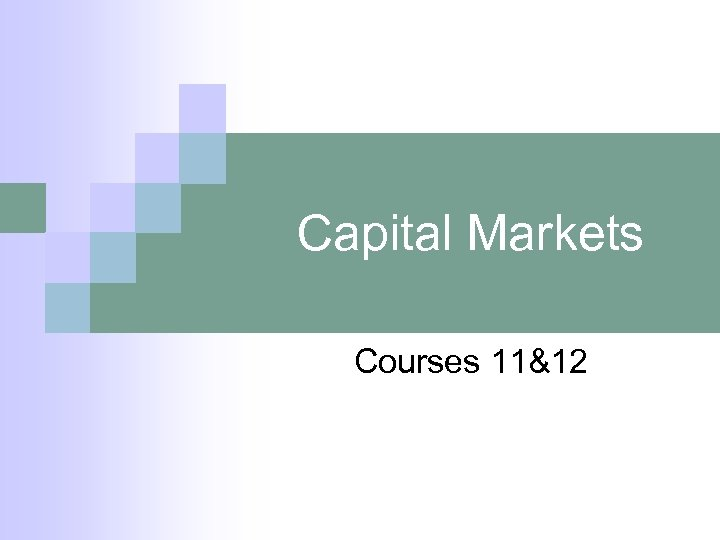Capital Markets Courses 11&12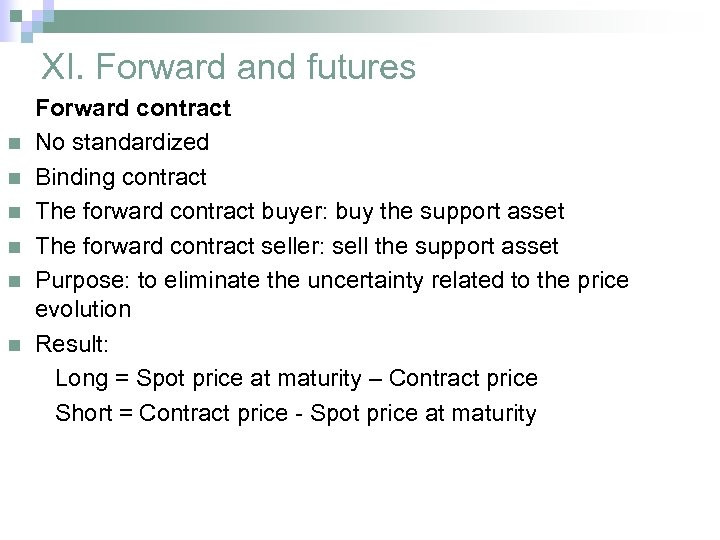XI. Forward and futures n n n Forward contract No standardized Binding contract The forward contract buyer: buy the support asset The forward contract seller: sell the support asset Purpose: to eliminate the uncertainty related to the price evolution Result: Long = Spot price at maturity – Contract price Short = Contract price - Spot price at maturity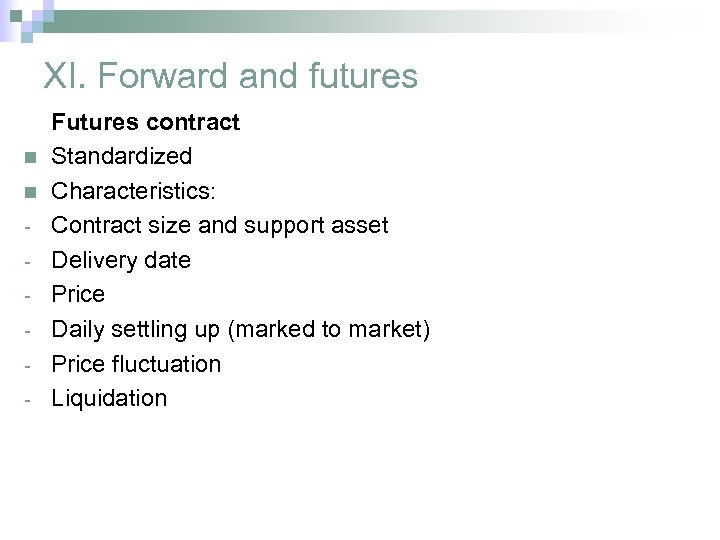XI. Forward and futures n n - Futures contract Standardized Characteristics: Contract size and support asset Delivery date Price Daily settling up (marked to market) Price fluctuation Liquidation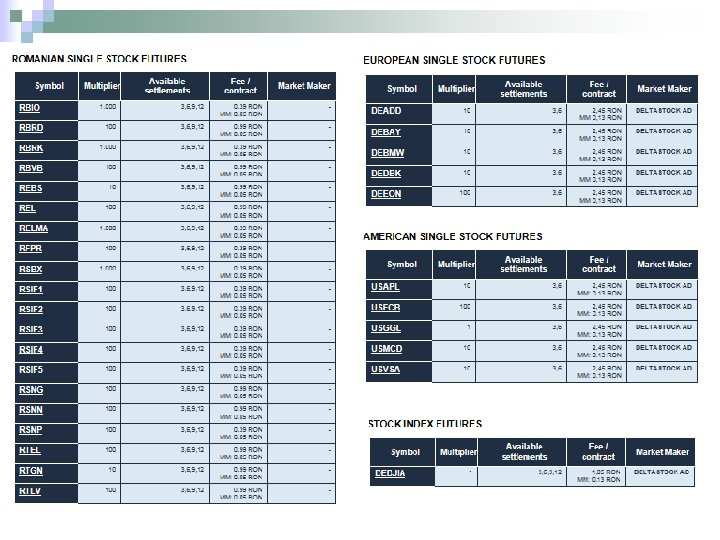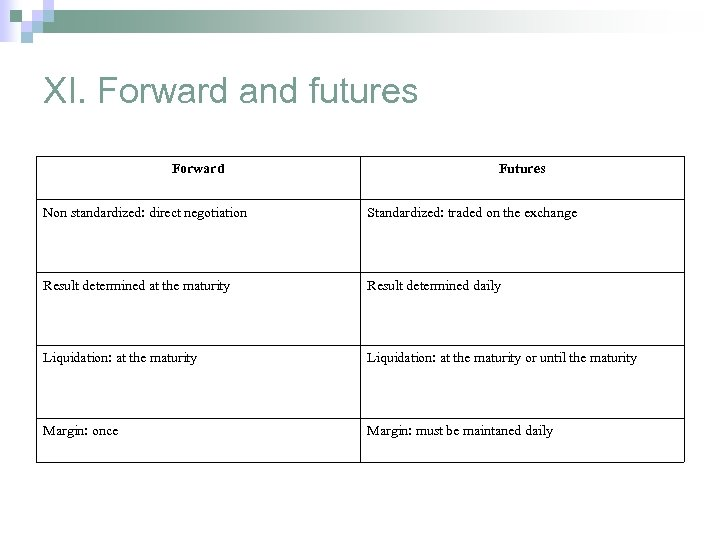XI. Forward and futures Forward Futures Non standardized: direct negotiation Standardized: traded on the exchange Result determined at the maturity Result determined daily Liquidation: at the maturity or until the maturity Margin: once Margin: must be maintaned daily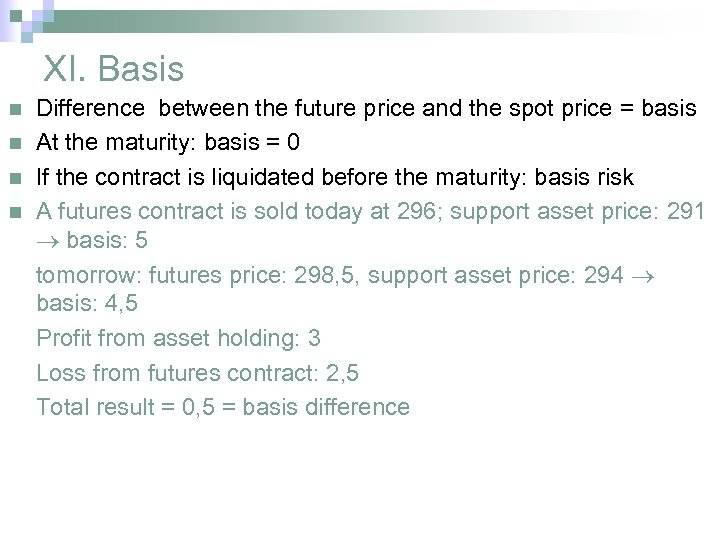XI. Basis n n Difference between the future price and the spot price = basis At the maturity: basis = 0 If the contract is liquidated before the maturity: basis risk A futures contract is sold today at 296; support asset price: 291 basis: 5 tomorrow: futures price: 298, 5, support asset price: 294 basis: 4, 5 Profit from asset holding: 3 Loss from futures contract: 2, 5 Total result = 0, 5 = basis difference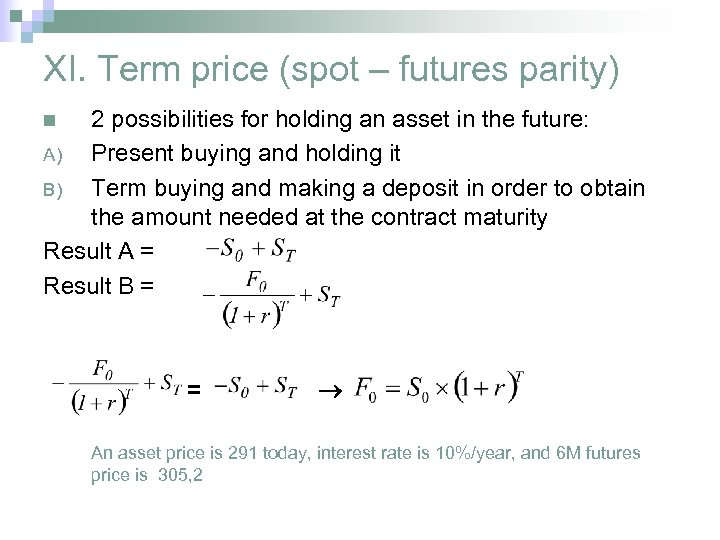XI. Term price (spot – futures parity) 2 possibilities for holding an asset in the future: A) Present buying and holding it B) Term buying and making a deposit in order to obtain the amount needed at the contract maturity Result A = Result B = n = An asset price is 291 today, interest rate is 10%/year, and 6 M futures price is 305, 2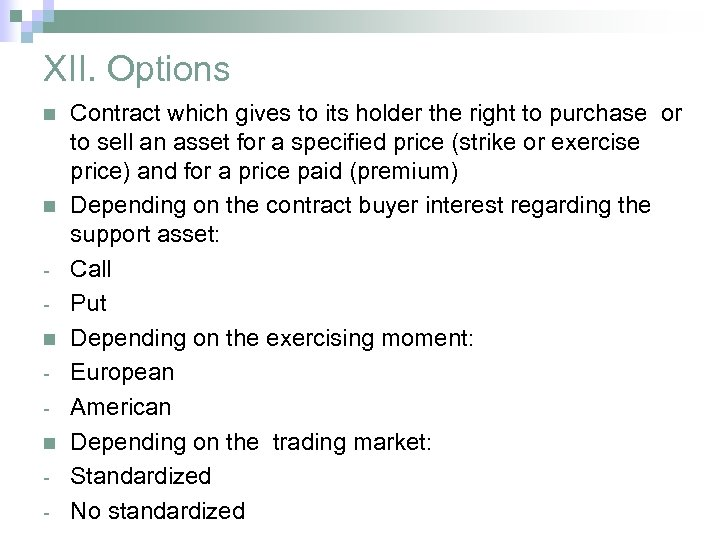XII. Options n n - Contract which gives to its holder the right to purchase or to sell an asset for a specified price (strike or exercise price) and for a price paid (premium) Depending on the contract buyer interest regarding the support asset: Call Put Depending on the exercising moment: European American Depending on the trading market: Standardized No standardized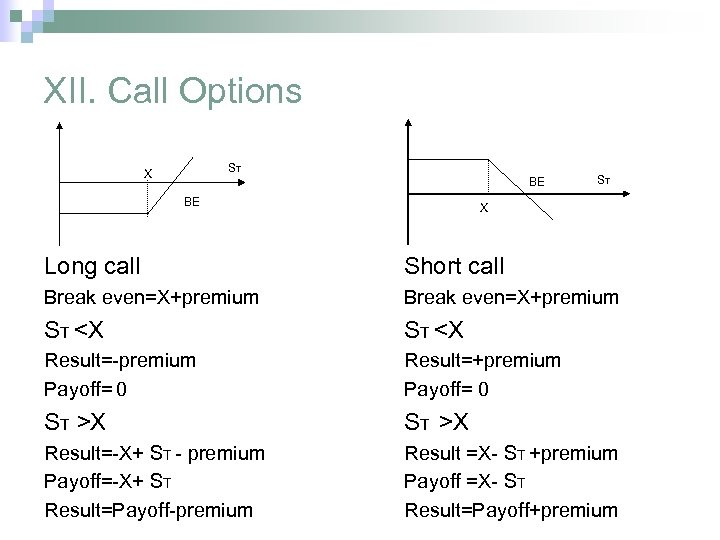XII. Call Options ST X BE BE ST X Long call Short call Break even=X+premium ST X Result=-X+ ST - premium Payoff=-X+ ST Result=Payoff-premium Result =X- ST +premium Payoff =X- ST Result=Payoff+premium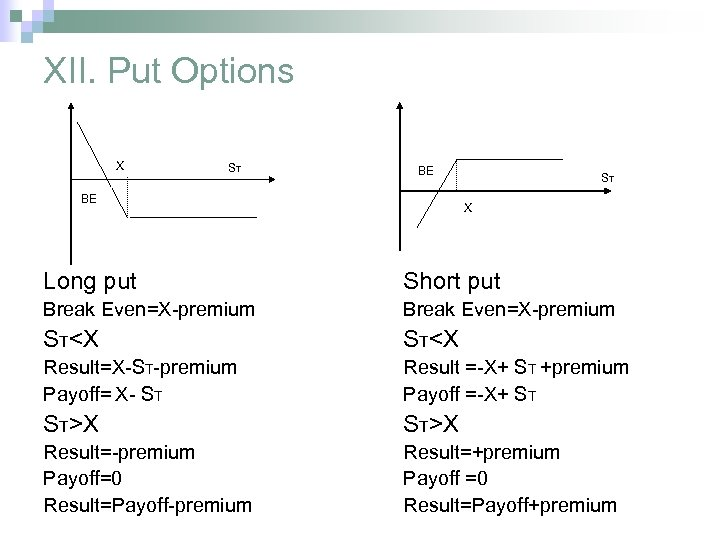XII. Put Options X ST BE BE ST X Long put Short put Break Even=X-premium STX Result=-premium Payoff=0 Result=Payoff-premium Result=+premium Payoff =0 Result=Payoff+premium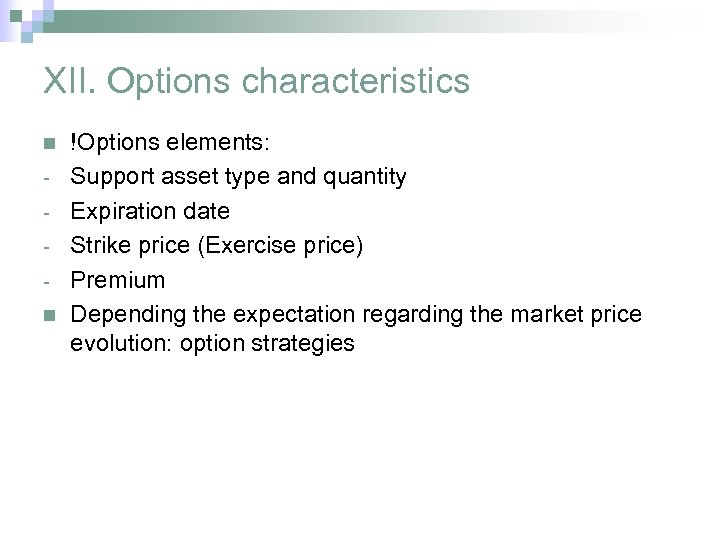XII. Options characteristics n n !Options elements: Support asset type and quantity Expiration date Strike price (Exercise price) Premium Depending the expectation regarding the market price evolution: option strategies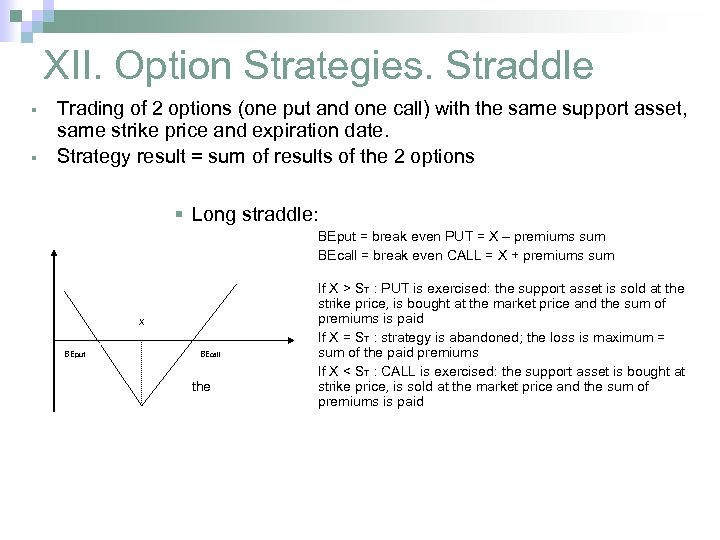XII. Option Strategies. Straddle § § Trading of 2 options (one put and one call) with the same support asset, same strike price and expiration date. Strategy result = sum of results of the 2 options § Long straddle: BEput = break even PUT = X – premiums sum BEcall = break even CALL = X + premiums sum X BEput BEcall the If X > ST : PUT is exercised: the support asset is sold at the strike price, is bought at the market price and the sum of premiums is paid If X = ST : strategy is abandoned; the loss is maximum = sum of the paid premiums If X < ST : CALL is exercised: the support asset is bought at strike price, is sold at the market price and the sum of premiums is paid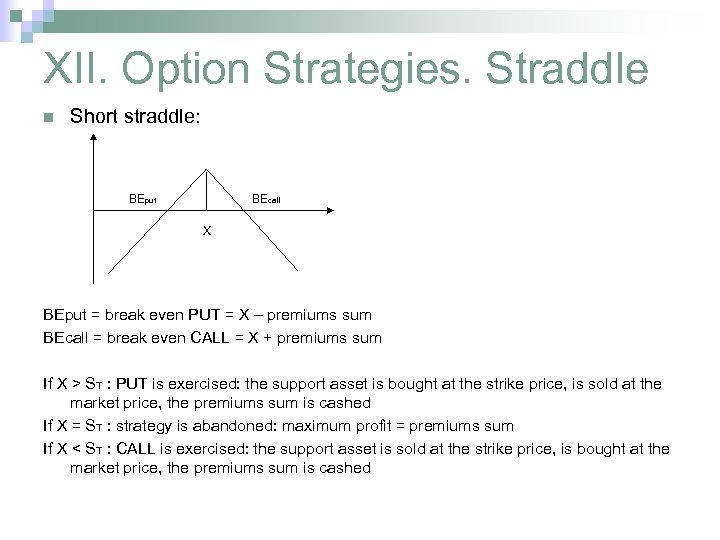XII. Option Strategies. Straddle n Short straddle: BEput BEcall X BEput = break even PUT = X – premiums sum BEcall = break even CALL = X + premiums sum If X > ST : PUT is exercised: the support asset is bought at the strike price, is sold at the market price, the premiums sum is cashed If X = ST : strategy is abandoned: maximum profit = premiums sum If X < ST : CALL is exercised: the support asset is sold at the strike price, is bought at the market price, the premiums sum is cashed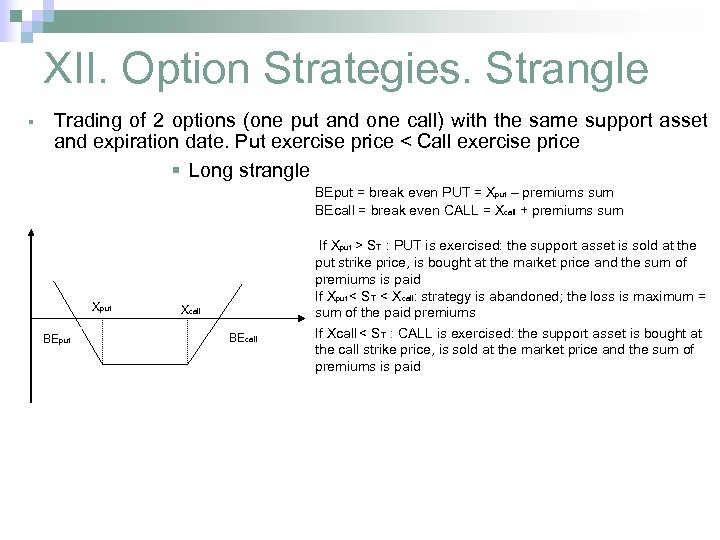XII. Option Strategies. Strangle § Trading of 2 options (one put and one call) with the same support asset and expiration date. Put exercise price < Call exercise price § Long strangle BEput = break even PUT = Xput – premiums sum BEcall = break even CALL = Xcall + premiums sum Xput BEput Xcall BEcall If Xput > ST : PUT is exercised: the support asset is sold at the put strike price, is bought at the market price and the sum of premiums is paid If Xput < ST < Xcall: strategy is abandoned; the loss is maximum = sum of the paid premiums If Xcall < ST : CALL is exercised: the support asset is bought at the call strike price, is sold at the market price and the sum of premiums is paid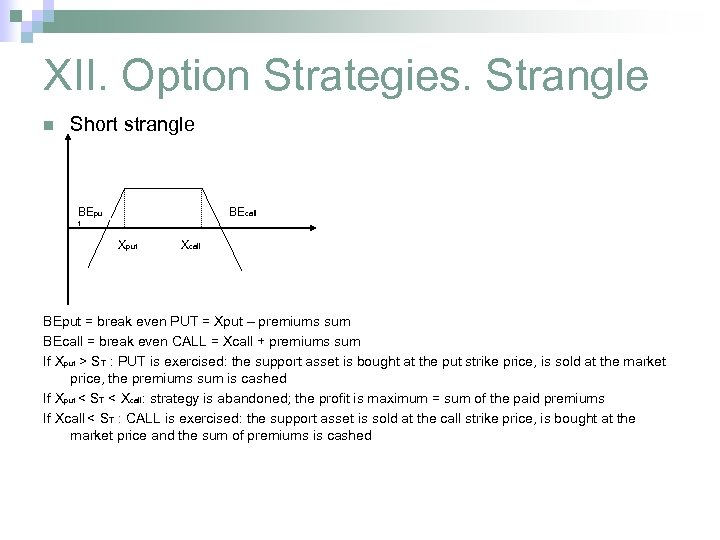XII. Option Strategies. Strangle n Short strangle BEpu BEcall t Xput Xcall BEput = break even PUT = Xput – premiums sum BEcall = break even CALL = Xcall + premiums sum If Xput > ST : PUT is exercised: the support asset is bought at the put strike price, is sold at the market price, the premiums sum is cashed If Xput < ST < Xcall: strategy is abandoned; the profit is maximum = sum of the paid premiums If Xcall < ST : CALL is exercised: the support asset is sold at the call strike price, is bought at the market price and the sum of premiums is cashed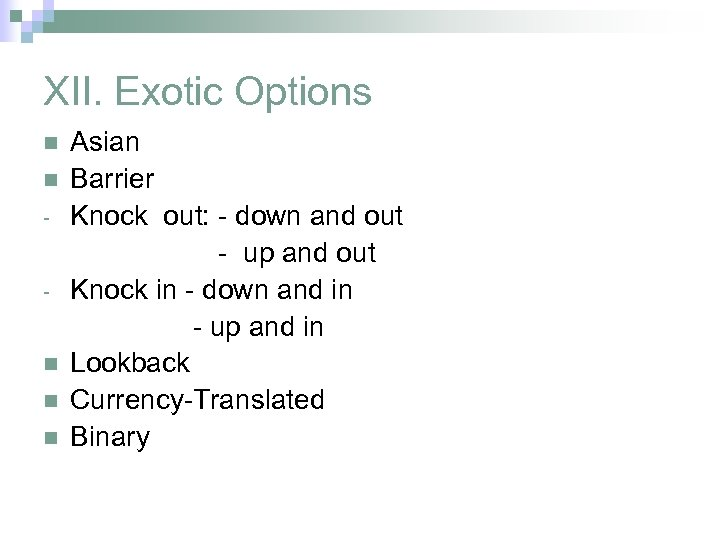XII. Exotic Options n n n Asian Barrier Knock out: - down and out - up and out Knock in - down and in - up and in Lookback Currency-Translated Binary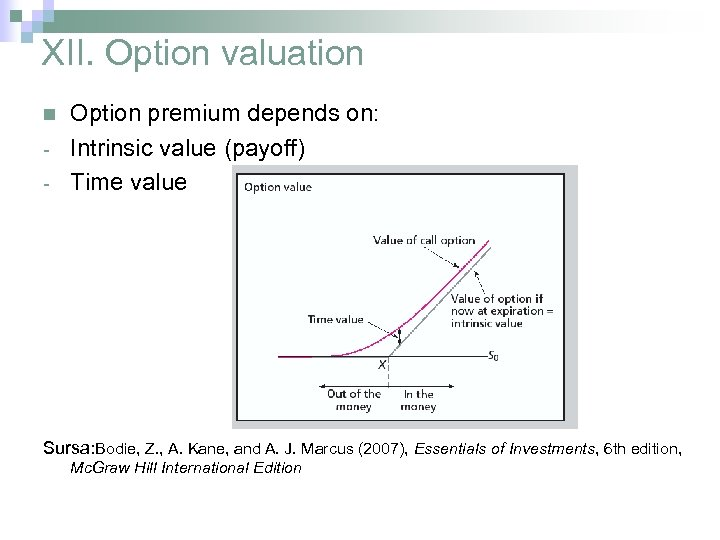XII. Option valuation n - Option premium depends on: Intrinsic value (payoff) Time value Sursa: Bodie, Z. , A. Kane, and A. J. Marcus (2007), Essentials of Investments, 6 th edition, Mc. Graw Hill International Edition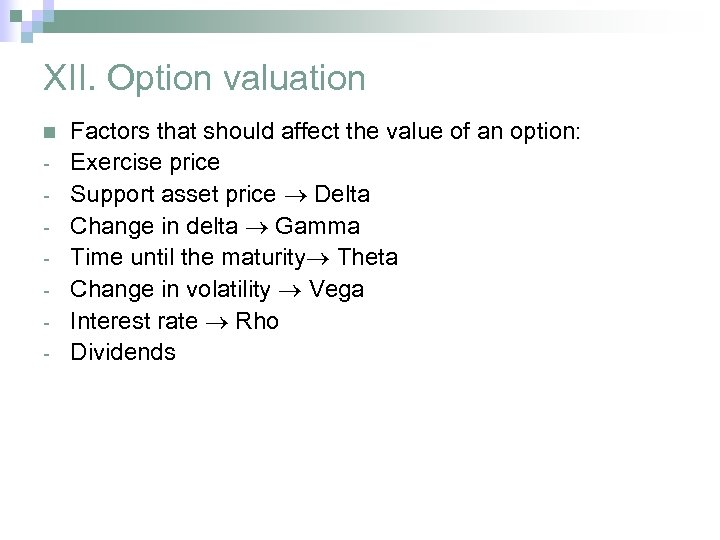XII. Option valuation n - Factors that should affect the value of an option: Exercise price Support asset price Delta Change in delta Gamma Time until the maturity Theta Change in volatility Vega Interest rate Rho Dividends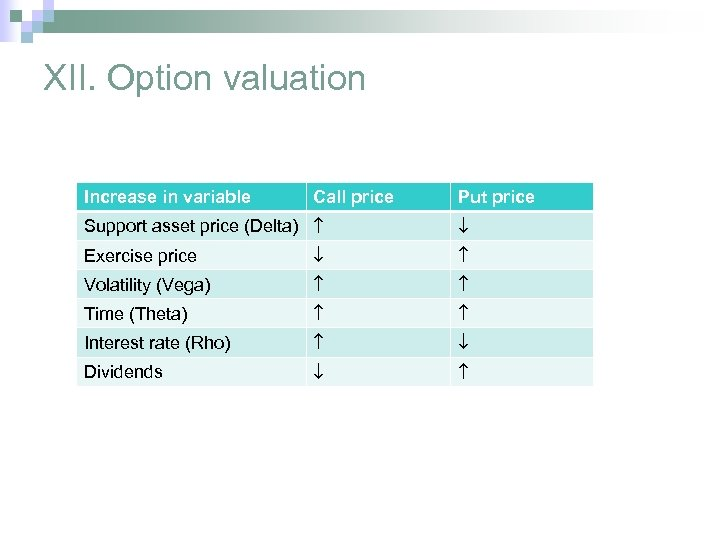XII. Option valuation Increase in variable Call price Put price Support asset price (Delta) Exercise price Volatility (Vega) Time (Theta) Interest rate (Rho) Dividends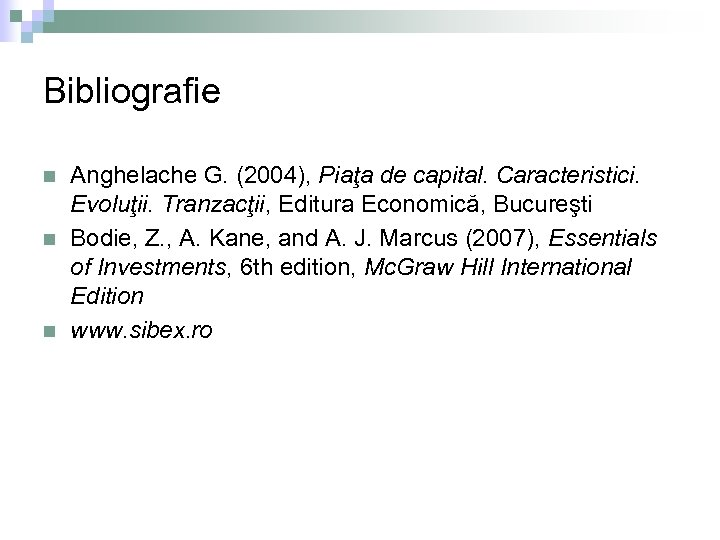Bibliografie n n n Anghelache G. (2004), Piaţa de capital. Caracteristici. Evoluţii. Tranzacţii, Editura Economică, Bucureşti Bodie, Z. , A. Kane, and A. J. Marcus (2007), Essentials of Investments, 6 th edition, Mc. Graw Hill International Edition www. sibex. ro